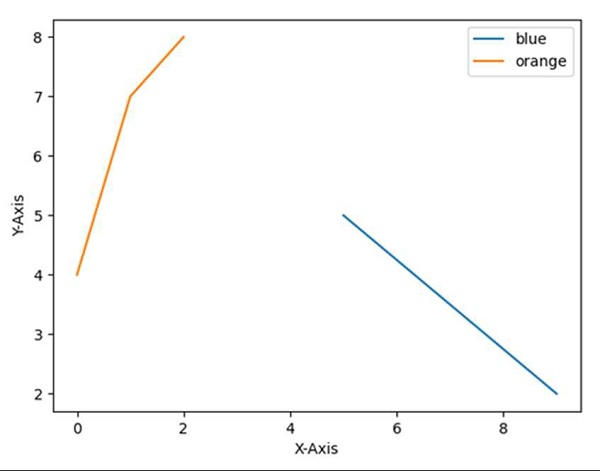# Remove or adapt the border of the frame of legend using matplotlib

To remove or adapt the border of the frame of legend we can follow the following steps −

• Set the X-axis label using the plt.xlabel() method.

• Set the Y-axis label using the plt.ylabel() method.

• Plot the lines using the plt.plot() method with [9, 5], [2, 5] and [4, 7, 8] array.

• Initializing two variables; location = 0 for the best location and border_drawn_flag = True (True, if border to be drawn for legend. False, if border is not drawn).

• Use the plt.legend() method for the legend and set the location and border_drawn_flag accordingly to get the perfect legend in the diagram.

• plt.show() method would help to show the figure.

## Example

import matplotlib.pyplot as plt

plt.ylabel("Y-axis ")
plt.xlabel("X-axis ")

plt.plot([9, 5], [2, 5], [4, 7, 8])

location = 0       # For the best location
border_drawn_flag = True
plt.legend(["blue", "orange"], loc=0, frameon=border_drawn_flag)

plt.show()

## OutputUpdated on: 15-Mar-2021

801 Views